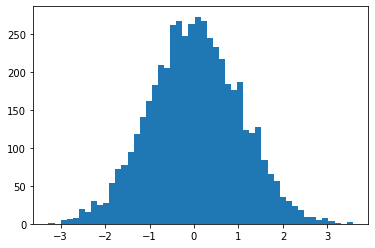# NumPy basicsNumPy is the primary matrix laboratory for Python. Many other libraries such as pandas, tensorflow, scikit-learn etc are built on top of this. Some tutorials to go with this cheat sheet: Numpy quick start - scipy, numpy python course eu, datacamp numpy wiki, numpy.org

In :
import numpy


### Creating numpy arrays from lists¶¶

In :
l1 = [1,2,3]
arr1 = numpy.array(l1)
arr1

Out:
array([1, 2, 3])
In :
type(arr1)

Out:
numpy.ndarray

#### 2D arrays¶¶

Create using list of lists

In :
l2d = [[1,2,3],[4,5,6]]
arr2d = numpy.array(l2d)
arr2d

Out:
array([[1, 2, 3],
[4, 5, 6]])
In :
type(arr2d)

Out:
numpy.ndarray

#### 3D arrays¶¶

Same way using list of lists

In :
l3d = [[[1,2],[3,4]], [[1,2],[3,4]]]
arr3d = numpy.array(l3d)
arr3d

Out:
array([[[1, 2],
[3, 4]],

[[1, 2],
[3, 4]]])

#### Creating using MATLAB style syntax¶¶

In :
mat = numpy.mat('1,2,3;4,5,6;7,8,9')
type(mat)

Out:
numpy.matrix
In :
mat

Out:
matrix([[1, 2, 3],
[4, 5, 6],
[7, 8, 9]])
In :
numpy.array(mat)

Out:
array([[1, 2, 3],
[4, 5, 6],
[7, 8, 9]])

### Special numpy arrays¶¶

#### ones zeros and eye¶¶

Creating a matrix of ones

In :
numpy.ones(shape=(4,4)) #pass shapre as a tuple

Out:
array([[ 1.,  1.,  1.,  1.],
[ 1.,  1.,  1.,  1.],
[ 1.,  1.,  1.,  1.],
[ 1.,  1.,  1.,  1.]])
##### zeros¶¶

sometimes it is useful to just get a matrix of zeros

In :
numpy.zeros(shape = (2,2))

Out:
array([[ 0.,  0.],
[ 0.,  0.]])
##### eye¶¶

eye for identity matrix - has values only in the diagonal. Identity matrices are square, 2D

In :
numpy.eye(3)

Out:
array([[ 1.,  0.,  0.],
[ 0.,  1.,  0.],
[ 0.,  0.,  1.]])

#### arange and linspace¶¶

arange is array range. Works same as range function in Python, but returns an array.

In :
numpy.arange(start=0, stop=10, step=1) #stop is non inclusive

Out:
array([0, 1, 2, 3, 4, 5, 6, 7, 8, 9])
In :
numpy.arange(21) #the stop arg is the only compulsory arg

Out:
array([ 0,  1,  2,  3,  4,  5,  6,  7,  8,  9, 10, 11, 12, 13, 14, 15, 16,
17, 18, 19, 20])

linspace is similar, returns contiguous numbers in linearly spaced intervals. These numbers conform to uniform distribution

In :
numpy.linspace(start=3, stop=21, num=7) #return 7 numbers between 3 and 21 (inclusive)

Out:
array([  3.,   6.,   9.,  12.,  15.,  18.,  21.])

#### Random numbers¶¶

generate random numbers using random module

##### rand¶¶

returns numbers in normal distribution between -1 and 1

In :
numpy.random.rand(3, 3) #specify shape in individual arguments

Out:
array([[0.13036297, 0.44737951, 0.85299833],
[0.94987992, 0.63348932, 0.42257387],
[0.54558662, 0.17654393, 0.84926165]])
In :
numpy.random.rand(3,3,3)

Out:
array([[[0.30709651, 0.26703385, 0.56179506],
[0.00490574, 0.79481741, 0.08887725],
[0.01482835, 0.41434266, 0.6668987 ]],

[[0.68209787, 0.9032678 , 0.87111643],
[0.83040063, 0.40690954, 0.43069501],
[0.44060348, 0.6184007 , 0.43669199]],

[[0.25732679, 0.90222633, 0.86477316],
[0.59079063, 0.23197552, 0.73234759],
[0.02420268, 0.58581809, 0.04223459]]])

randn Create random numbers that follow standard normal distribution.

In :
vals = numpy.random.randn(5000)
vals.shape

Out:
(5000,)
In :
import matplotlib.pyplot as plt
%matplotlib inline
plt.hist(vals, bins=50);##### randint¶¶

randint returns randomly distributed integers between specified range

In :
numpy.random.randint(low=30, high=200, size=10)

Out:
array([ 37,  43,  76,  36,  67,  45, 165,  75, 165,  40])

### Array inspection¶¶

#### shape¶¶

Use shape property to get the dimensions

In :
arr2 = numpy.random.rand(3,3,3)
arr2.shape

Out:
(3, 3, 3)
In :
arr3 = numpy.random.randint(low=30, high=200, size=10)
arr3.shape

Out:
(10,)

thus shape is returned as a tuple.

#### datatype of the elements¶¶

In :
arr2.dtype

Out:
dtype('float64')
In :
arr3.dtype

Out:
dtype('int32')

#### max and min elements¶¶

In :
arr2

Out:
array([[[ 0.04539372,  0.63141413,  0.89693763],
[ 0.55265413,  0.16925386,  0.83917698],
[ 0.75055999,  0.26155305,  0.33921729]],

[[ 0.35913789,  0.20122447,  0.10491535],
[ 0.01784351,  0.20815688,  0.90825816],
[ 0.69680734,  0.3975908 ,  0.63961161]],

[[ 0.06461796,  0.99271516,  0.02077921],
[ 0.26578436,  0.40538054,  0.58002467],
[ 0.53456854,  0.85680407,  0.66601052]]])
In :
arr2.max() #max element in the entire 3d array

Out:
0.99271516081323574
In :
arr2.max(axis=1) #max in each column

Out:
array([[ 0.75055999,  0.63141413,  0.89693763],
[ 0.69680734,  0.3975908 ,  0.90825816],
[ 0.53456854,  0.99271516,  0.66601052]])
In :
arr2.max(axis=2) #max in each row

Out:
array([[ 0.89693763,  0.83917698,  0.75055999],
[ 0.35913789,  0.90825816,  0.69680734],
[ 0.99271516,  0.58002467,  0.85680407]])

min method to find the minimum

In :
arr2.min()

Out:
0.017843505898512246
In :
arr2.min(axis=1)

Out:
array([[ 0.04539372,  0.16925386,  0.33921729],
[ 0.01784351,  0.20122447,  0.10491535],
[ 0.06461796,  0.40538054,  0.02077921]])
##### argmax and argmin to find the location of max element¶¶
In :
arr2.argmax() #location max element in the 3D array

Out:
19
In :
arr2.argmax(1) #indices of each max element

Out:
array([[2, 0, 0],
[2, 2, 1],
[2, 0, 2]], dtype=int64)
In :
arr2.argmin(1)

Out:
array([[0, 1, 2],
[1, 0, 0],
[0, 1, 0]], dtype=int64)

### Array manipulation¶¶

#### Reshape arrays¶¶

reshape() method on an array object and send a tuple of the rows and column dimensions

In :
arr3

Out:
array([145, 152, 101, 130, 152,  84, 148, 160, 121, 137])
In :
arr3.shape

Out:
(10,)
In :
arr3.reshape((5,2))

Out:
array([[145, 152],
[101, 130],
[152,  84],
[148, 160],
[121, 137]])